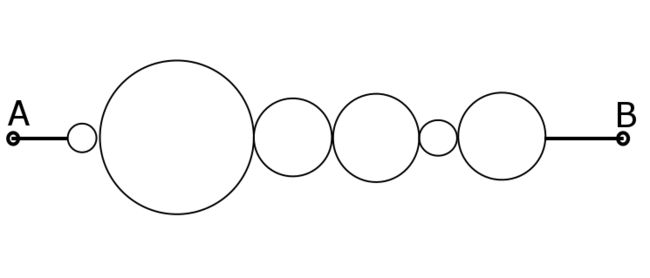Resistance of ringsA wire of resistance $R$ and length $l$ is divided into six rings of radius in ratio $1:2:3:4:5:6$. The resistance between two terminals is of the form $\frac{a}{b}R$, find $a+b$.

×